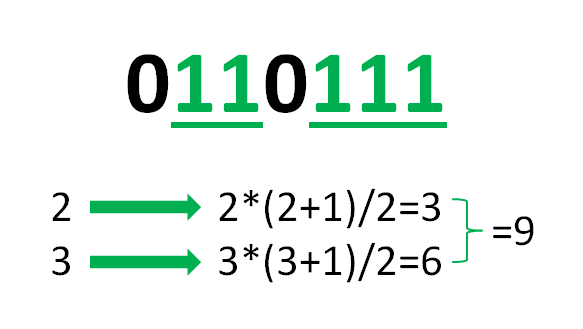Open in App
Not now

# Count of substrings containing only the given character

• Last Updated : 10 May, 2021

Given a string S and a character C, the task is to count the number of substrings of S that contains only the character C.
Examples:

Input: S = “0110111”, C = ‘1’
Output:
Explanation:
The substrings containing only ‘1’ are:
“1” — 5 times
“11” — 3 times
“111” — 1 time
Hence, the count is 9.

Input: S = “geeksforgeeks”, C = ‘e’
Output:

Naive Approach:
The simplest approach is to generate all possible substrings of the given string S and count the substrings which contains only character C.
Time Complexity: O(N3
Space Complexity: O(1)
Efficient Approach:
To optimize the above approach, the fact that a string of length N forms N*(N+1)/2 substrings can be applied. Therefore, for N consecutive occurrence of character C in the string, N*(N+1)/2 substrings are generated. Hence, iterate through the entire length of the string S and for each consecutive substring of character C, count the possible number of substrings possible from them and add to the total count of substrings possible.

Illustration:
S = “0110111”, C = ‘1’Below is the implementation of the above approach:

## C++

 `// C++ program to implement``// the above approach``#include ``using` `namespace` `std;` `// Function that finds the count``// of substrings containing only``// character C in the string S``void` `countSubString(string S, ``char` `C)``{``    ``// To store total count``    ``// of substrings``    ``int` `count = 0;` `    ``// To store count of``    ``// consecutive C's``    ``int` `conCount = 0;` `    ``// Loop through the string``    ``for` `(``char` `ch : S) {` `        ``// Increase the consecutive``        ``// count of C's``        ``if` `(ch == C)``            ``conCount++;` `        ``else` `{` `            ``// Add count of sub-strings``            ``// from consecutive strings``            ``count += (conCount``                      ``* (conCount + 1))``                     ``/ 2;` `            ``// Reset the consecutive``            ``// count of C's``            ``conCount = 0;``        ``}``    ``}` `    ``// Add count of sub-strings from``    ``// consecutive strings``    ``count += (conCount``              ``* (conCount + 1))``             ``/ 2;` `    ``// Print the count of sub-strings``    ``// containing only C``    ``cout << count;``}` `// Driver Code``int` `main()``{``    ``string S = ``"geeksforgeeks"``;` `    ``char` `C = ``'e'``;` `    ``countSubString(S, C);` `    ``return` `0;``}`

## Java

 `// Java program for the above approach``import` `java.util.*;` `class` `GFG{``    ` `// Function that finds the count``// of substrings containing only``// character C in the string S``static` `void` `countSubString(String S, ``char` `C)``{``    ` `    ``// To store total count``    ``// of substrings``    ``int` `count = ``0``;` `    ``// To store count of``    ``// consecutive C's``    ``int` `conCount = ``0``;` `    ``// Loop through the string``    ``for``(``int` `i = ``0``; i < S.length(); i++)``    ``{``        ``char` `ch = S.charAt(i);``        ` `        ``// Increase the consecutive``        ``// count of C's``        ``if` `(ch == C)``            ``conCount++;` `        ``else``        ``{``            ` `            ``// Add count of sub-strings``            ``// from consecutive strings``            ``count += (conCount *``                     ``(conCount + ``1``)) / ``2``;` `            ``// Reset the consecutive``            ``// count of C's``            ``conCount = ``0``;``        ``}``    ``}` `    ``// Add count of sub-strings from``    ``// consecutive strings``    ``count += (conCount *``             ``(conCount + ``1``)) / ``2``;` `    ``// Print the count of sub-strings``    ``// containing only C``    ``System.out.println(count);``}` `// Driver Code``public` `static` `void` `main(String s[])``{``    ``String S = ``"geeksforgeeks"``;``    ``char` `C = ``'e'``;``    ` `    ``countSubString(S, C);``}``}` `// This code is contributed by rutvik_56`

## Python3

 `# Python3 program to implement``# the above approach` `# Function that finds the count``# of substrings containing only``# character C in the S``def` `countSubString(S, C):` `    ``# To store total count``    ``# of substrings``    ``count ``=` `0` `    ``# To store count of``    ``# consecutive C's``    ``conCount ``=` `0` `    ``# Loop through the string``    ``for` `ch ``in` `S:` `        ``# Increase the consecutive``        ``# count of C's``        ``if` `(ch ``=``=` `C):``            ``conCount ``+``=` `1``            ` `        ``else``:` `            ``# Add count of sub-strings``            ``# from consecutive strings``            ``count ``+``=` `((conCount ``*``                      ``(conCount ``+` `1``)) ``/``/` `2``)` `            ``# Reset the consecutive``            ``# count of C's``            ``conCount ``=` `0` `    ``# Add count of sub-strings from``    ``# consecutive strings``    ``count ``+``=` `((conCount ``*``              ``(conCount ``+` `1``)) ``/``/` `2``)` `    ``# Print the count of sub-strings``    ``# containing only C``    ``print``(count)` `# Driver Code``if` `__name__ ``=``=` `'__main__'``:``  ` `    ``S ``=` `"geeksforgeeks"``    ``C ``=` `'e'` `    ``countSubString(S, C)``    ` `# This code is contributed by mohit kumar 29`

## C#

 `// C# program for the above approach ``using` `System;` `class` `GFG{` `// Function that finds the count``// of substrings containing only``// character C in the string S``static` `void` `countSubString(String S, ``char` `C)``{` `    ``// To store total count``    ``// of substrings``    ``int` `count = 0;` `    ``// To store count of``    ``// consecutive C's``    ``int` `conCount = 0;` `    ``// Loop through the string``    ``for``(``int` `i = 0; i < S.Length; i++)``    ``{``        ``char` `ch = S[i];` `        ``// Increase the consecutive``        ``// count of C's``        ``if` `(ch == C)``            ``conCount++;` `        ``else``        ``{``            ` `            ``// Add count of sub-strings``            ``// from consecutive strings``            ``count += (conCount *``                     ``(conCount + 1)) / 2;` `            ``// Reset the consecutive``            ``// count of C's``            ``conCount = 0;``        ``}``    ``}` `    ``// Add count of sub-strings from``    ``// consecutive strings``    ``count += (conCount *``             ``(conCount + 1)) / 2;` `    ``// Print the count of sub-strings``    ``// containing only C``    ``Console.Write(count);``}` `// Driver Code``public` `static` `void` `Main(String[] args)``{``    ``String S = ``"geeksforgeeks"``;``    ``char` `C = ``'e'``;` `    ``countSubString(S, C);``}``}` `// This code is contributed by grand_master`

## Javascript

 ``

Output:

`6`

Time Complexity: O(N)
Auxiliary Space: O(1)

My Personal Notes arrow_drop_up